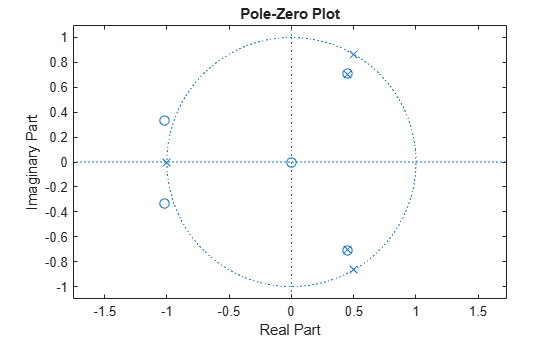# invfreqz

Identify discrete-time filter parameters from frequency response data

## Syntax

``[b,a] = invfreqz(h,w,n,m)``
``[b,a] = invfreqz(h,w,n,m,wt)``
``[b,a] = invfreqz(___,iter)``
``[b,a] = invfreqz(___,tol)``
``[b,a] = invfreqz(___,'trace')``
``[b,a] = invfreqz(h,w,'complex',n,m,___)``

## Description

````[b,a] = invfreqz(h,w,n,m)` returns the real numerator and denominator coefficient vectors `b` and `a` of the transfer function `h`.```
````[b,a] = invfreqz(h,w,n,m,wt)` weights the fit-errors versus frequency using `wt`.```

example

````[b,a] = invfreqz(___,iter)` provides an algorithm that guarantees stability of the resulting linear system by searching for the best fit using a numerical, iterative scheme. This syntax can include any combination of input arguments from the previous syntaxes.```
````[b,a] = invfreqz(___,tol)` uses `tol` to decide convergence of the iterative algorithm.```
````[b,a] = invfreqz(___,'trace')` displays a textual progress report of the iteration.```
````[b,a] = invfreqz(h,w,'complex',n,m,___)` creates a complex filter. In this case no symmetry is enforced, and the frequency is specified in radians between –π and π.```

## Examples

collapse all

Convert a simple transfer function to frequency response data and then back to the original filter coefficients. Sketch the zeros and poles of the function.

```a = [1 2 3 2 1 4]; b = [1 2 3 2 3]; [h,w] = freqz(b,a,64); [bb,aa] = invfreqz(h,w,4,5)```
```bb = 1×5 1.0000 2.0000 3.0000 2.0000 3.0000 ```
```aa = 1×6 1.0000 2.0000 3.0000 2.0000 1.0000 4.0000 ```
`zplane(bb,aa)``bb` and `aa` are equivalent to `b` and `a`, respectively. However, the system is unstable because it has poles outside the unit circle. Use `invfreqz`'s iterative algorithm to find a stable approximation to the system. Verify that the poles are within the unit circle.

`[bbb,aaa] = invfreqz(h,w,4,5,[],30)`
```bbb = 1×5 0.2427 0.2788 0.0069 0.0971 0.1980 ```
```aaa = 1×6 1.0000 -0.8944 0.6954 0.9997 -0.8933 0.6949 ```
`zplane(bbb,aaa)`## Input Arguments

collapse all

Frequency response, specified as a vector.

Angular frequencies at which `h` is computed, specified as a vector.

Desired order of the numerator and denominator polynomials, specified as positive integer scalars.

Weighting factors, specified as a vector. `wt` is a vector of weighting factors that is the same length as `w`.

Number of iterations in the search algorithm, specified as a positive real scalar. The `iter` parameter tells `invfreqz` to end the iteration when the solution has converged, or after `iter` iterations, whichever comes first.

Tolerance, specified as a scalar. `invfreqz` defines convergence as occurring when the norm of the (modified) gradient vector is less than `tol`.

To obtain a weight vector of all ones, use

`invfreqz(h,w,n,m,[],iter,tol)`

## Output Arguments

collapse all

Transfer function coefficients, returned as vectors. Express the transfer function in terms of `b` and `a` as

`$H\left(s\right)=\frac{B\left(s\right)}{A\left(s\right)}=\frac{b\left(1\right){s}^{n}+b\left(2\right){s}^{n-1}+\cdots +b\left(n+1\right)}{a\left(1\right){s}^{m}+a\left(2\right){s}^{m-1}+\cdots +a\left(m+1\right)}$`

Example: `b = [1 3 3 1]/6` and `a = [3 0 1 0]/3` specify a third-order Butterworth filter with normalized 3 dB frequency 0.5π rad/sample.

Data Types: `double` | `single`
Complex Number Support: Yes

## Algorithms

By default, `invfreqz` uses an equation error method to identify the best model from the data. This finds `b` and `a` in

`$\underset{b,a}{\mathrm{min}}\sum _{k=1}^{n}wt\left(k\right){|h\left(k\right)A\left(w\left(k\right)\right)-B\left(w\left(k\right)\right)|}^{2}$`

by creating a system of linear equations and solving them with the MATLAB® `\` operator. Here A(ω(k)) and B(ω(k)) are the Fourier transforms of the polynomials `a` and `b`, respectively, at the frequency ω(k), and n is the number of frequency points (the length of `h` and `w`). This algorithm is a based on Levi .

The superior (“output-error”) algorithm uses the damped Gauss-Newton method for iterative search , with the output of the first algorithm as the initial estimate. This solves the direct problem of minimizing the weighted sum of the squared error between the actual and the desired frequency response points.

`$\underset{b,a}{\mathrm{min}}\sum _{k=1}^{n}wt\left(k\right){|h\left(k\right)-\frac{B\left(w\left(k\right)\right)}{A\left(w\left(k\right)\right)}|}^{2}$`

 Levi, E. C. “Complex-Curve Fitting.” IRE Transactions on Automatic Control. Vol. AC-4, 1959, pp. 37–44.

 Dennis, J. E., Jr., and R. B. Schnabel. Numerical Methods for Unconstrained Optimization and Nonlinear Equations. Englewood Cliffs, NJ: Prentice-Hall, 1983.